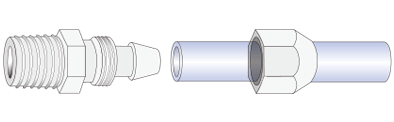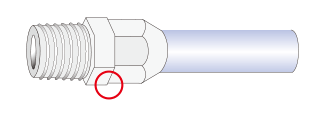### Matching Evaluation Test Data

 Model Number with Fitting Model Burst Pressure Pull-Out Strength (N) Air Leak Test 20$^\circ$C 60$^\circ$C 20$^\circ$C 20$^\circ$C$\times$0.2MPa (2Bar) E-SJ-2$\times$4E-SJ-2$\times$4-CBU E-SJ-2$\times$4 AL-010401CP 7.5MPa (75Bar) 4.1MPa (41Bar) 146 No Leaking E-KYT-2$\times$4 2.7MPa (27Bar) 1.5MPa(15Bar) 72 E-SJUS-2$\times$4 E-SJUS-2$\times$4-BK 3.8MPa (38Bar) 2.3MPa(23Bar) 62 E-SJ-4$\times$6E-SJ-4$\times$6-CBU E-SJ-4$\times$6-BK AL-010602CP 4.2MPa (42Bar) 2.5MPa(25Bar) 268 E-SJUS-4$\times$6 E-SJUS-4$\times$6-BK 2.1MPa (21Bar) 1.2MPa (12Bar) 123 E-KYT-4$\times$6 1.5MPa (15Bar) 0.8MPa (8Bar) 98 E-SJ-6$\times$8E-SJ-6$\times$8-CBU E-SJ-6$\times$8-BK AL-010802CP 3.1MPa (31Bar) 1.7MPa (17Bar) 339 E-SJUS-6$\times$8 E-SJUS-6$\times$8-BK 1.5MPa (15Bar) 0.8MPa (8Bar) 173 E-KYT-6$\times$8 1.1MPa (11Bar) 0.55MPa (5.5Bar) 121

Cautions:

1. These data are test values and not guaranteed values. Please make a judgment on your own when you use this product.
2. The burst test values by temperature are burst pressure data, not working pressure. Check the working pressure of each tube for safety use.
3. The pull strength is the value at the time when the tube which pulled the joint came off from the joint or the tube was torn at 200 mm/min with the joint in the tube.
4. In the air tightness test, after 30 minutes of condition adjustment with the joint set, 0.2MPa (2Bar) of air is filled in for 30 minutes, and it is submerged to check if there is any air leak near the joint. E-KYT-6 x 8 was conducted at 0.15MPa (1.5Bar).

### Chemical Resistant Data

Chemical Name
AL Fittings (PEEK)
E-FTS (SUS316L)
E-SJ (ETFE)
E-KYT (TPO)
Acetic Acid (30%)$\bigcirc$$\triangle$$\bigcirc$$\bigcirc Sulfuric Acid (40%)\bigcirc$$\times$$\bigcirc$$\bigcirc$
Chromic Acid (40%)$\bigcirc$-$\bigcirc$$\times Methanol\bigcirc$$\triangle$$\bigcirc$$\bigcirc$
Ethanol$\bigcirc$$\bigcirc$$\bigcirc$$\bigcirc Ethylene Glycol\bigcirc$$\triangle$$\bigcirc$$\bigcirc$
Acetaldehyde$\bigcirc$$\bigcirc$$\bigcirc$$\triangle Acetone\bigcirc$$\triangle$$\bigcirc$$\bigcirc$
Methyl Ethyl Ketone$\bigcirc$$\triangle$$\bigcirc$$\times N-Methyl-2-Pyrrolidone\bigcirc-\bigcirc- Ammonia Water (10%)\bigcirc$$\triangle$$\bigcirc- Ethyl Acetate\bigcirc$$\triangle$$\bigcirc$$\triangle$
Diethyl Ether$\bigcirc$$\triangle$$\bigcirc$-
Tetrahydrofuran$\bigcirc$$\bigcirc$$\bigcirc$$\bigcirc Chloroform\bigcirc$$\triangle$$\bigcirc$$\triangle$
Benzene$\bigcirc$$\triangle$$\bigcirc$$\triangle Toluene\bigcirc$$\bigcirc$$\bigcirc$$\triangle$
Gasoline$\bigcirc$$\bigcirc$$\bigcirc$$\times$

Cautions: This chemical resistance data is for reference and does not guarantee the actual use. Even though the result shows ○, it may not be suitable, depending on the environment, conditions, period and so on. Please evaluate the chemical matching on your own.

### How to Assemble Fittings

(A) Cut the tubing in a perpendicular way. Pass a nut through the tubing.(B) Insert the nipple to the tubing. Make sure to insert to the end. There should be no gap between tubing and fittings.(C) Tighten the nut by wrench until there is no gap between the fitting body and the fitting nut.Assembly Done !